# Xenon tetroxide (XeO4) lewis structure, molecular geometry, hybridization, polar or nonpolar

Home  > Chemistry Article > XeO4 lewis structure and its molecular geometry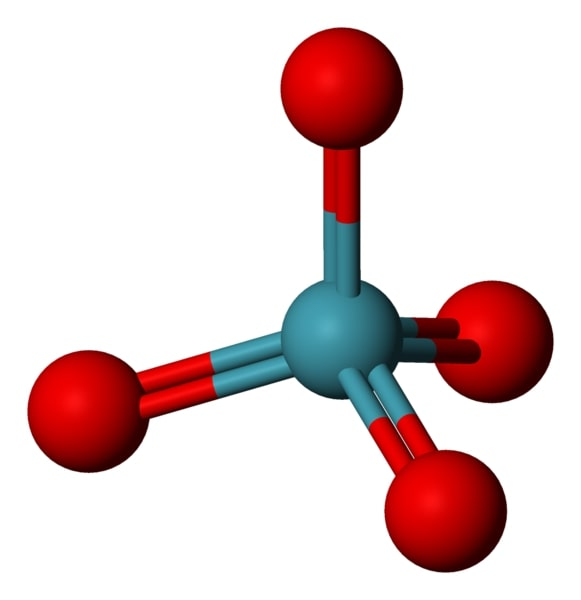Xenon tetroxide appears as a yellow crystalline solid with molecular formula XeO4. The temperature above -36 °C, it is more prone to explode and scatter into elemental xenon and oxygen.

In this tutorial, we will discuss Xenon tetroxide (XeO4) lewis structure, molecular geometry, hybridization, polar or nonpolar, etc.

Xenon tetroxide is a powerful explosive at temperatures above −35.9 °C. Its boiling point is 0 °C and its melting point is −35.9 °C.

 Name of Molecule Xenon tetroxide Chemical formula XeO4 Molecular geometry of XeO4 Tetrahedral Electron geometry of XeO4 Tetrahedral Hybridization Sp3 Nature Nonpolar molecule Total Valence electron for XeO4 32
Page Contents

## How to draw lewis structure of XeO4?

XeO4 lewis structure is made up of one xenon and four oxygen atom, the xenon is in a central position, and all oxygen is at the surrounding position. There are four double bonds(Xe=O) present in the XeO4 lewis structure.

The lewis structure of XeO4 contains 8 pairs of nonbonding electrons and 8 pairs of bonding electrons.

## Follow some steps for drawing the Lewis structure for XeO4

1. Count total valence electron in XeO4

First of all, determine the valence electron that is available for drawing the lewis structure of XeO4. To determine the valence electron, look at the group number of atoms in the periodic table.

The xenon atom belongs to group 18th in the periodic table and oxygen is situated in the 16th group, hence, the valence electron for xenon is 8 and for oxygen atom, it is 6.

⇒ Total number of the valence electrons in xenon = 8

⇒ Total number of the valence electrons in oxygen = 6

∴ Total number of valence electron available for the XeO4 Lewis structure = 8 + 6(4) = 32 valence electrons         [∴XeO4 molecule has one xenon and four oxygen atoms]2. Find the least electronegative atom and place it at center

An atom with a less electronegative value is more preferable for the central position in the lewis diagram because they are more prone to share the electrons with surrounding atoms.

In the case of the XeO4 molecule, the electronegativity for the xenon atom is 2.6 and for the oxygen atom, it is 3.44.

Therefore, place the xenon atom at the central position and all oxygen atoms at the surrounding position.3. Connect outer atoms to central atom with a single bond

In this step, place the single bond in between the outer atoms(oxygen) and central atom(xenon).After connecting each outer atom to the central atom, count the number of valence electrons used in the above structure. There are 4 single used in the above structure, and one single bond means 2 electrons.

Hence, in the above structure, (4 × 2) = 8 valence electrons are used from a total of 32 valence electrons available for drawing the XeO4 Lewis structure.

∴ (32 – 8) = 24 valence electrons

So, we are left with 24 valence electrons more.

4. Place remaining electrons on outer atoms and complete their octet

Let’s start putting the remaining valence electrons on outer atoms first. In the case of the XeO4 molecule, oxygen is the outer atom and it needs 8 electrons in the valence shell to complete the octet.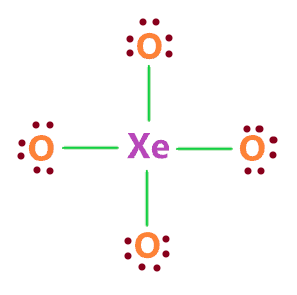So, all oxygen atoms in the above structure completed their octet, because all of them have 8 electrons(6 electrons represented as dots + 2 electrons in every single bond) in their valence shell.

Also, the central atom Xenon has also 8 electrons(4 single bonds) in its valence shell.

Now again count the number of valence electrons used in the above structure.

In the above structure, there are 24 electrons represented as dots + 4 single bonds means 8 electrons.

Hence, (24 + 8) = 32 total electrons are used in the above structure and we had a total of 32 valence electrons available for the XeO4 lewis structure.

Now we have to determine the formal charge for the above structure to check whether it is stable or not.

5. Check the stability with the help of a formal charge concept

The lesser the formal charge on atoms, the better is the stability of the lewis diagram.

To calculate the formal charge on an atom. Use the formula given below-

⇒ Formal charge = (valence electrons – lone pair electrons –  1/2 bonding electrons)

Let’s count the formal charge on the oxygen atom first, all oxygen atoms in the XeO4 Lewis structure(4th step) have the same bonded pair and lone pair, so, just count the F.C. for the one oxygen atom.

For oxygen atom:

⇒ Valence electrons of oxygen = 6

⇒ Lone pair electrons on oxygen = 6

⇒ Bonding electrons around oxygen(1 single bond) = 2

∴ (6 – 6 – 2/2) = -1 formal charge on each oxygen atom.

For xenon atom

⇒ Valence electrons of xenon = 8

⇒ Lone pair electrons on xenon = 0

⇒ Bonding electrons around xenon (4 single bonds) = 8

∴ (8 – 0 – 8/2) = +4 formal charge on the xenon central atom.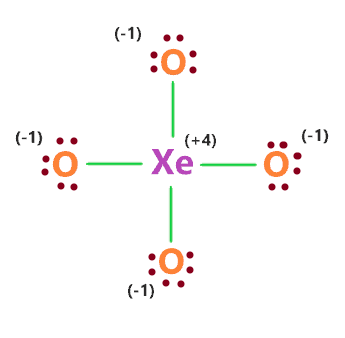The above XeO4 lewis structure is not stable because of the high formal charge. To reduce this, we have to rearrange the electrons and form multiple bonds.

All you have to do is to convert the one lone pair of each oxygen atom to a bond pair.

The central atom Xenon has expanded octet that means it can hold more than 8 electrons in its valence shell.

Xenon atom has d-orbital for extra electrons needed for bonding.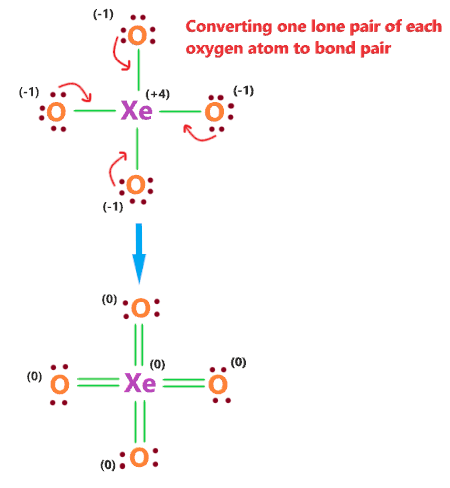Now you can calculate the formal charge for each atom in the above structure, you will get the formal charge for every atom equal to zero.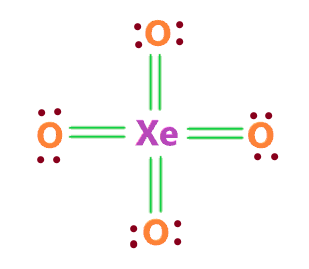### XeO4 lewis structure

That’s all this is our most stable and appropriate lewis structure of XeO4.

## What is the molecular geometry of XeO4?

The molecular geometry of XeO4 is Tetrahedral since the central atom xenon is attached to four oxygen atoms and it contains no lone pair, so, the region of electron density around the xenon atom is 4 and all the regions are bonding region that implies its shape will be tetrahedral in nature.

Double bonds will be counted as one region of electron density.

According to the VSEPR theory, “The maximum distance four regions of electron density can get away from affords a geometry called tetrahedral.”## Hybridization of XeO4

Let’s find the hybridization of XeO4 through the steric number of its central atom.

“Steric number is the addition of a total number of bonded atoms around a central atom and the lone pair present on it.”

∴ Steric number of XeO4 = (Number of bonded atoms attached to xenon + Lone pair on xenon)

As per the lewis structure of XeO4, the xenon atom is bonded with four oxygen atoms and it contains no lone pair of electrons.

∴ Steric number of XeO4 = (4 + 0) = 4

 Steric number Hybridization 1 S 2 Sp 3 Sp² 4 Sp³ 5 Sp³d 6 Sp³d²

So, for a steric number of four, we get the Sp3 hybridization on the xenon atom in the XeO4 molecule.

## Xenon tetroxide polarity: is XeO4 polar or nonpolar?

Is XeO4 polar or non-polar? XeO4 is a non-polar molecule because it contains four bonds(Xe=O) that are arranged symmetrically to each other in tetrahedral shapes. Due to this, the dipole moment generated along these will cancel out each other making it’s a non-polar molecule.

The net dipole moment of XeO4 is zero.

## FAQ

### Why does Xenon in XeO4 lewis structure violate octet and holds more than 8 electrons?

In the XeO4 lewis structure, the central atom is xenon which is surrounded by 4 double bonds means 16 bonding electrons, hence, its valence shell is holding more than 8 electrons which violates the octet.

“The octet rule refers to the tendency of atoms to prefer to have eight electrons in the valence shell”.

Now the xenon atom in the XeO4 lewis structure violates the octet because it has the ability to expand the octet basically called an expanded octet.

The xenon atom has a d-orbital to store the extra electrons needed for bonding. In the XeO4 lewis structure, the xenon atom has to hold more than 8 electrons for reducing the formal charge and attaining stability.

At last, we can say, the xenon atom is holding more than 8 electrons because of obtaining the most stable lewis structure of XeO4 by using its ability of expanded octet.

### How many bonding and nonbonding pairs of electrons are present in the lewis structure of XeO4?

The bonding electrons are those which form a bond and are basically found in between two atoms. The nonbonding electrons are those which do not take part in bonding, basically called lone pairs and represented as dots in the lewis diagram.

Now in the XeO4 lewis structure, there are 16 nonbonding electrons(represented as dots) and 16 bonding electrons(4 double bonds).

So, the pairs of bonding electrons are 8, and pairs of nonbonding electrons are also 8 in the XeO4 lewis structure.

Properties of Xenon tetroxide

• It has a molar mass of 195.29 g mol−1
• It is explosive.
• It appears as a Yellow solid below −36°C.
• It has a melting point of −35.9 °C and a boiling point of 0 ºC.

Reactions of Xenon tetroxide

Dissolving xenon tetroxide in water forms perxenic acid.

⇒ XeO4 + 2 H2O → H4XeO6

In alkalis, xenon tetroxide forms perxenate salts.

⇒ XeO4 + 4 NaOH → Na4XeO6 + 2 H2O

In reaction with xenon hexafluoride, xenon tetroxide gives xenon oxyfluorides.

⇒ XeO4 + XeF6 → XeOF4 + XeO3F2

## Summary

• The total valence electron is available for drawing the XeO4 Lewis structure is 32.
• The steric number of Xenon central atom in the XeO4 molecule is 4, thus, it forms Sp3 hybridization.
• The net dipole moment of XeO4 is zero, hence, it is a nonpolar molecule.
• The molecular geometry of XeO4 is tetrahedral because the central atom Xenon is surrounded by 4 regions of electron density.
• The electron geometry of XeO4 is also tetrahedral because the central atom Xenon has no lone pair which can influence it, hence, its molecular geometry and electron geometry are the same.
• Each oxygen atom in the XeO4 lewis structure has 4 electrons that do not involve in bonding.
##### Subscribe to Blog via Email

Join 2 other subscribers

Share it...